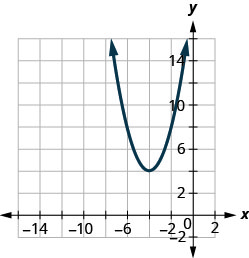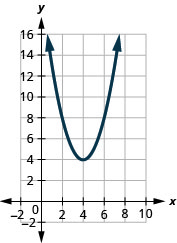# 9.8E：练习

•• OpenStax
• OpenStax
$$\newcommand{\vecs}{\overset { \rightharpoonup} {\mathbf{#1}} }$$ $$\newcommand{\vecd}{\overset{-\!-\!\rightharpoonup}{\vphantom{a}\smash {#1}}}$$$$\newcommand{\id}{\mathrm{id}}$$ $$\newcommand{\Span}{\mathrm{span}}$$ $$\newcommand{\kernel}{\mathrm{null}\,}$$ $$\newcommand{\range}{\mathrm{range}\,}$$ $$\newcommand{\RealPart}{\mathrm{Re}}$$ $$\newcommand{\ImaginaryPart}{\mathrm{Im}}$$ $$\newcommand{\Argument}{\mathrm{Arg}}$$ $$\newcommand{\norm}{\| #1 \|}$$ $$\newcommand{\inner}{\langle #1, #2 \rangle}$$ $$\newcommand{\Span}{\mathrm{span}}$$ $$\newcommand{\id}{\mathrm{id}}$$ $$\newcommand{\Span}{\mathrm{span}}$$ $$\newcommand{\kernel}{\mathrm{null}\,}$$ $$\newcommand{\range}{\mathrm{range}\,}$$ $$\newcommand{\RealPart}{\mathrm{Re}}$$ $$\newcommand{\ImaginaryPart}{\mathrm{Im}}$$ $$\newcommand{\Argument}{\mathrm{Arg}}$$ $$\newcommand{\norm}{\| #1 \|}$$ $$\newcommand{\inner}{\langle #1, #2 \rangle}$$ $$\newcommand{\Span}{\mathrm{span}}$$$$\newcommand{\AA}{\unicode[.8,0]{x212B}}$$

### 练习成就完美

##### 练习$$\PageIndex{23}$$ Graph Quadratic Functions of the Form $$f(x)=x^{2}=k$$

1. 在同一个矩形坐标系上绘制二次函数的图形
2. 描述在函数中添加常量对基本抛物线有什么影响。$$k$$
1. $$f(x)=x^{2}, g(x)=x^{2}+4, \text { and } h(x)=x^{2}-4$$
2. $$f(x)=x^{2}, g(x)=x^{2}+7, \text { and } h(x)=x^{2}-7$$

1。

1.图 9.7.71
2. $$g(x)=x^{2}+4$$图形与单位的图形相同，$$f(x)=x^{2}$$$$4$$单位向上移动。 的图形$$h(x)=x^{2}-4$$与单位的图形相同，$$f(x)=x^{2}$$$$4$$单位向下移动。
##### 练习$$\PageIndex{24}$$ Graph Quadratic Functions of the Form $$f(x)=x^{2}=k$$

1. $$f(x)=x^{2}+3$$
2. $$f(x)=x^{2}-7$$
3. $$g(x)=x^{2}+2$$
4. $$g(x)=x^{2}+5$$
5. $$h(x)=x^{2}-4$$
6. $$h(x)=x^{2}-5$$

1。

3。

5。

##### 练习$$\PageIndex{25}$$ Graph Quadratic Functions of the Form $$f(x)=(x-h)^{2}$$

1. 在同一个矩形坐标系上绘制二次函数的图形
2. 描述在圆括号内添加常量会产生什么效果$$h$$
1. $$f(x)=x^{2}, g(x)=(x-3)^{2}, \text { and } h(x)=(x+3)^{2}$$
2. $$f(x)=x^{2}, g(x)=(x+4)^{2}, \text { and } h(x)=(x-4)^{2}$$

1。

1.图 9.7.75
2. 的图形$$g(x)=(x−3)^{2}$$与图形相同，$$f(x)=x^{2}$$$$3$$单位向右移动。 的图形$$h(x)=(x+3)^{2}$$与图形相同，$$f(x)=x^{2}$$但向左移动了$$3$$单位。
##### 练习$$\PageIndex{26}$$ Graph Quadratic Functions of the Form $$f(x)=(x-h)^{2}$$

1. $$f(x)=(x-2)^{2}$$
2. $$f(x)=(x-1)^{2}$$
3. $$f(x)=(x+5)^{2}$$
4. $$f(x)=(x+3)^{2}$$
5. $$f(x)=(x-5)^{2}$$
6. $$f(x)=(x+2)^{2}$$

1。

3。

5。

##### 练习$$\PageIndex{27}$$ Graph Quadratic Functions of the Form $$f(x)=(x-h)^{2}$$

1. $$f(x)=(x+2)^{2}+1$$
2. $$f(x)=(x+4)^{2}+2$$
3. $$f(x)=(x-1)^{2}+5$$
4. $$f(x)=(x-3)^{2}+4$$
5. $$f(x)=(x+3)^{2}-1$$
6. $$f(x)=(x+5)^{2}-2$$
7. $$f(x)=(x-4)^{2}-3$$
8. $$f(x)=(x-6)^{2}-2$$

1。

3。

5。

7。

##### 练习$$\PageIndex{28}$$ Graph Quadratic Functions of the Form $$f(x)=ax^{2}$$

1. $$f(x)=-2 x^{2}$$
2. $$f(x)=4 x^{2}$$
3. $$f(x)=-4 x^{2}$$
4. $$f(x)=-x^{2}$$
5. $$f(x)=\frac{1}{2} x^{2}$$
6. $$f(x)=\frac{1}{3} x^{2}$$
7. $$f(x)=\frac{1}{4} x^{2}$$
8. $$f(x)=-\frac{1}{2} x^{2}$$

1。

3。

5。

7。

##### 练习$$\PageIndex{29}$$ Graph Quadratic Functions Using Transformations

1. $$f(x)=-3 x^{2}-12 x-5$$
2. $$f(x)=2 x^{2}-12 x+7$$
3. $$f(x)=3 x^{2}+6 x-1$$
4. $$f(x)=-4 x^{2}-16 x-9$$

1。 $$f(x)=-3(x+2)^{2}+7$$

3。 $$f(x)=3(x+1)^{2}-4$$

##### 练习$$\PageIndex{30}$$ Graph Quadratic Functions Using Transformations

1. $$f(x)=a(x−h)^{2}+k$$表单重写每个函数
2. 使用转换将其绘制成图形
1. $$f(x)=x^{2}+6 x+5$$
2. $$(x)=x^{2}+4 x-12$$
3. $$f(x)=x^{2}+4 x-12$$
4. $$f(x)=x^{2}-6 x+8$$
5. $$f(x)=x^{2}-6 x+15$$
6. $$f(x)=x^{2}+8 x+10$$
7. $$f(x)=-x^{2}+8 x-16$$
8. $$f(x)=-x^{2}+2 x-7$$
9. $$f(x)=-x^{2}-4 x+2$$
10. $$f(x)=-x^{2}+4 x-5$$
11. $$f(x)=5 x^{2}-10 x+8$$
12. $$f(x)=3 x^{2}+18 x+20$$
13. $$f(x)=2 x^{2}-4 x+1$$
14. $$f(x)=3 x^{2}-6 x-1$$
15. $$f(x)=-2 x^{2}+8 x-10$$
16. $$f(x)=-3 x^{2}+6 x+1$$

1。

1. f (x) = (x+3) ^ {2} -4

3。

1. $$f(x)=(x+2)^{2}-1$$

5。

1. $$f(x)=(x-3)^{2}+6$$

7。

1. $$f(x)=-(x-4)^{2}+0$$

9。

1. $$f(x)=-(x+2)^{2}+6$$

11。

1. $$f(x)=5(x-1)^{2}+3$$

13。

1. $$f(x)=2(x-1)^{2}-1$$

15。

1. $$f(x)=-2(x-2)^{2}-2$$
##### 练习$$\PageIndex{31}$$ Graph Quadratic Functions Using Transformations

1. $$f(x)=a(x−h)^{2}+k$$表单重写每个函数
2. 使用属性绘制图表
1. $$f(x)=2 x^{2}+4 x+6$$
2. $$f(x)=3 x^{2}-12 x+7$$
3. $$f(x)=-x^{2}+2 x-4$$
4. $$f(x)=-2 x^{2}-4 x-5$$

1。

1. $$f(x)=2(x+1)^{2}+4$$

3。

1. $$f(x)=-(x-1)^{2}-3$$
##### 练习$$\PageIndex{32}$$ Matching

1. $$f(x)=x^{2}+4$$
2. $$f(x)=x^{2}-4$$
3. $$f(x)=(x+4)^{2}$$
4. $$f(x)=(x-4)^{2}$$
5. $$f(x)=(x+4)^{2}-4$$
6. $$f(x)=(x+4)^{2}+4$$
7. $$f(x)=(x-4)^{2}-4$$
8. $$f(x)=(x-4)^{2}+4$$

1.图 9.7.97

2.图 9.7.98

3.图 9.7.99

4.图 9.7.100

5.图 9.7.101

6.图 9.7.102

7.图 9.7.103

8.图 9.7.104

1. c

3. e

5. d

7.g

##### 练习$$\PageIndex{33}$$ Find a Quadratic Function from its Graph

1.图 9.7.105

2.图 9.7.106

3.图 9.7.107

4.图 9.7.108

1。 $$f(x)=(x+1)^{2}-5$$

3。 $$f(x)=2(x-1)^{2}-3$$

##### 练习$$\PageIndex{34}$$ Writing Exercise
1. 像我们在上一节中所做的那样，$$f(x)=x^{2}+4x+5$$首先使用属性绘制二次函数的图形，然后使用转换将其绘制成图形。 你更喜欢哪种方法？ 为什么？
2. 像我们在上一节中所做的那样，$$f(x)=2x^{2}−4x−3$$首先使用属性绘制二次函数的图形，然后使用转换将其绘制成图形。 你更喜欢哪种方法？ 为什么？

1。 答案可能有所不同。

## 自检

a. 完成练习后，使用此清单评估您对本节目标的掌握程度。